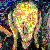### COSC 85/185 - Novel Frameworks in Theoretical Computer Science

(This course was taught in the spring term of 1996.)

 We explored novel frameworks in theoretical Computer Science by learning the basics about one or two results in several of the following areas (as time and the interests of the class dictate). In each case, the intention was to explore a particular framework or way of thinking that leads to interesting results."But what... is it good for!?"

Computing with DNA
Can test tubes of DNA be used to solve medium-size instances of NP-hard problems such as satisfiability?
On-Line Algorithms
We discussed the buy-or-rent problem (and how it relates to the k-server problem) on-line list management, and routing in networks.
Probabilistically Checkable Proofs and Hardness of Approximation
A "probabilistically checkable proof" requires only a constant number of bits (chosen at random) of the proof to be checked to convince the reader of its soundness. We explored how such proofs are possible and how their existence implies the NP-hardness of approximating many problems.
Greedy Combinatorial Approximation Algorithms by Probabilistic Methods
Randomized rounding is a general tool for deriving approximation algorithms for combinatorial optimization problems. We explored the method and a new technique for making especially efficient algorithms by this method. These algorithms are useful for solving large "packing and covering" linear programs.
Quantum Computation
We studied Shor's algorithm to factor numbers in polynomial time under a theoretical model of computation consistent with quantum mechanics. Follow the link to find references and an on-line tutorial on the algorithm.

Student projects:
Quantum cryptography! A tutorial by Jamie Ford and an on-line interactive demo by Fred Henle.
DNA computing bibliography, by Dax Burkhart.
A description of networks in practice by Omar Lari.
Departmental course description.